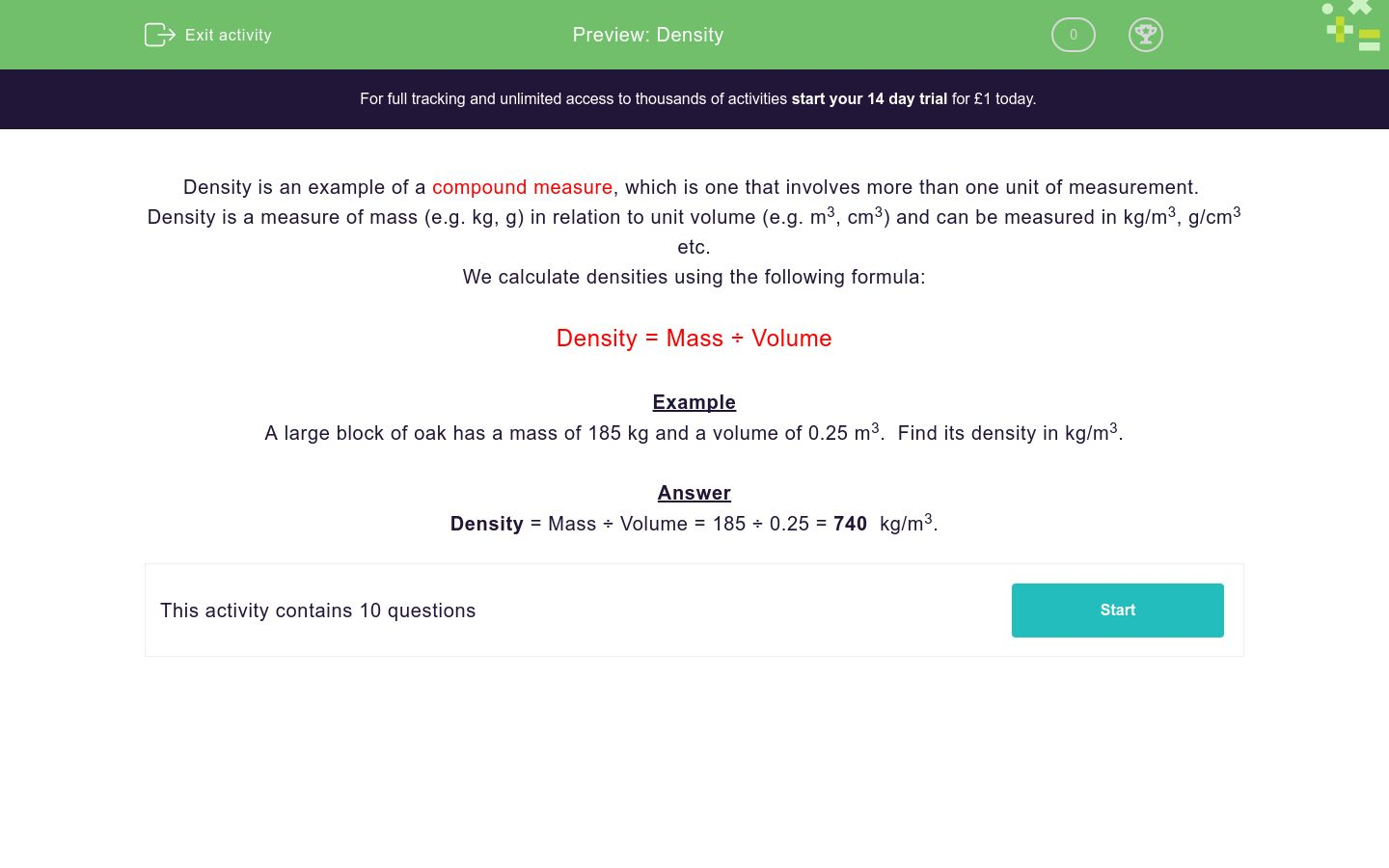# Density

In this worksheet, students work out densities given the mass and volume of objects.Key stage:  KS 4

Curriculum topic:  Ratio, Proportion and Rates of Change

Curriculum subtopic:  Convert Between Related Compound Units

Difficulty level:### QUESTION 1 of 10

Density is an example of a compound measure, which is one that involves more than one unit of measurement.

Density is a measure of mass (e.g. kg, g) in relation to unit volume (e.g. m3, cm3) and can be measured in kg/m3, g/cm3 etc.

We calculate densities using the following formula:

Density = Mass ÷ Volume

Example

A large block of oak has a mass of 185 kg and a volume of 0.25 m3.  Find its density in kg/m3.

Answer

Density = Mass ÷ Volume = 185 ÷ 0.25 = 740  kg/m3.

A large block of walnut wood has a mass of 171 kg and a volume of 0.3 m3

Find its density in kg/m3.

(just write the number)

A rock has a mass of 1.8 kg and a volume of 72 cm3

Find its density in g/cm3.

(just write the number)

A piece of charcoal has a mass of 10.4 g and a volume of 50 cm3

Find its density in g/cm3.

(just write the number)

A lump of butter has a mass of 250 g and a volume of 290 cm3

Find its density in g/cm3.

(just write the number to 3 sig. figs.)

A litre of water has a mass of 1 kg.

Find its density in g/cm3.

(just write the number)

A kilogram of lead has a volume of 88.18 cm3.

Find its density in g/cm3.

(just write the number to 2 dp)

A toy has a mass of 250 g and a volume of 1000 cm3.

Find its density in g/cm3.

(just write the number)

A marble statue has a mass of 3200 kg and a volume of 1.25 m3.

Find its density in kg/m3.

(just write the number)

A large slab of concrete has a mass of 24 kg and a volume of 0.01 m3.

Find its density in kg/m3.

(just write the number)

1 kg of flour has a volume of 1700 cm3.

Find its density in g/cm3.

(just write the number to 3 sig. figs.)

• Question 1

A large block of walnut wood has a mass of 171 kg and a volume of 0.3 m3

Find its density in kg/m3.

(just write the number)

CORRECT ANSWER
570
EDDIE SAYS
171 ÷ 0.3
• Question 2

A rock has a mass of 1.8 kg and a volume of 72 cm3

Find its density in g/cm3.

(just write the number)

CORRECT ANSWER
25
EDDIE SAYS
1800 ÷ 72
• Question 3

A piece of charcoal has a mass of 10.4 g and a volume of 50 cm3

Find its density in g/cm3.

(just write the number)

CORRECT ANSWER
0.208
EDDIE SAYS
10.4 ÷ 50
• Question 4

A lump of butter has a mass of 250 g and a volume of 290 cm3

Find its density in g/cm3.

(just write the number to 3 sig. figs.)

CORRECT ANSWER
0.862
EDDIE SAYS
250 ÷ 290
• Question 5

A litre of water has a mass of 1 kg.

Find its density in g/cm3.

(just write the number)

CORRECT ANSWER
1
EDDIE SAYS
1000 ÷ 1000
• Question 6

A kilogram of lead has a volume of 88.18 cm3.

Find its density in g/cm3.

(just write the number to 2 dp)

CORRECT ANSWER
11.34
EDDIE SAYS
1000 ÷ 88.18
• Question 7

A toy has a mass of 250 g and a volume of 1000 cm3.

Find its density in g/cm3.

(just write the number)

CORRECT ANSWER
0.25
EDDIE SAYS
250 ÷ 1000
• Question 8

A marble statue has a mass of 3200 kg and a volume of 1.25 m3.

Find its density in kg/m3.

(just write the number)

CORRECT ANSWER
2560
EDDIE SAYS
3200 ÷ 1.25
• Question 9

A large slab of concrete has a mass of 24 kg and a volume of 0.01 m3.

Find its density in kg/m3.

(just write the number)

CORRECT ANSWER
2400
EDDIE SAYS
24 ÷ 0.01
• Question 10

1 kg of flour has a volume of 1700 cm3.

Find its density in g/cm3.

(just write the number to 3 sig. figs.)

CORRECT ANSWER
0.588
EDDIE SAYS
1000 ÷ 1700
---- OR ----

Sign up for a £1 trial so you can track and measure your child's progress on this activity.

### What is EdPlace?

We're your National Curriculum aligned online education content provider helping each child succeed in English, maths and science from year 1 to GCSE. With an EdPlace account you’ll be able to track and measure progress, helping each child achieve their best. We build confidence and attainment by personalising each child’s learning at a level that suits them.

Get started#### Similar activities you may like

Start your £1 trial today.
Subscribe from £10/month.